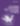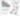# jQuery Convert RGB to Hex Colour Values

JavaScript

Share:

#### Free JavaScript Book!Write powerful, clean and maintainable JavaScript.

RRP \$11.95

Two JavaScript functions to convert rgb colour values to hex colour values and then second one converts hex colours values back to rgb. This is very useful for working with html colours and changing colours dynamically using jQuery.

## Function to Convert RGB to HEX

``````function rgb2hex(rgb){
rgb = rgb.match(/^rgb((d+),s*(d+),s*(d+))\$/);
return "#" +
("0" + parseInt(rgb,10).toString(16)).slice(-2) +
("0" + parseInt(rgb,10).toString(16)).slice(-2) +
("0" + parseInt(rgb,10).toString(16)).slice(-2);
}``````

### Function to Convert RGB to Hex (version 2)

``````function RGB2Color(r,g,b)
{
return '#' + this.byte2Hex(r) + this.byte2Hex(g) + this.byte2Hex(b);
}
function byte2Hex (n)
{
var nybHexString = "0123456789ABCDEF";
return String(nybHexString.substr((n >> 4) & 0x0F,1)) + nybHexString.substr(n & 0x0F,1);
}``````

## Function to Convert Hex to RGB

``````function hexToRgb(h)
{
var r = parseInt((cutHex(h)).substring(0,2),16), g = ((cutHex(h)).substring(2,4),16), b = parseInt((cutHex(h)).substring(4,6),16)
return r+''+b+''+b;
}
function cutHex(h) {return (h.charAt(0)=="#") ? h.substring(1,7):h}

//usage
console.log(hexToRgb("#FFFFFF"));``````

## Function to Convert HEX to RGB (PHP)

``````function hex2rgb( \$colour ) {
if ( \$colour == '#' ) {
\$colour = substr( \$colour, 1 );
}
if ( strlen( \$colour ) == 6 ) {
list( \$r, \$g, \$b ) = array( \$colour . \$colour, \$colour . \$colour, \$colour . \$colour );
} elseif ( strlen( \$colour ) == 3 ) {
list( \$r, \$g, \$b ) = array( \$colour . \$colour, \$colour . \$colour, \$colour . \$colour );
} else {
return false;
}
\$r = hexdec( \$r );
\$g = hexdec( \$g );
\$b = hexdec( \$b );
return array( 'red' => \$r, 'green' => \$g, 'blue' => \$b );
}``````

### Related Articles### Sam Deering

Sam Deering has 15+ years of programming and website development experience. He was a website consultant at Console, ABC News, Flight Centre, Sapient Nitro, and the QLD Government and runs a tech blog with over 1 million views per month. Currently, Sam is the Founder of Crypto News, Australia.

#### New books out now!Learn the basics of programming with the web's most popular language - JavaScript